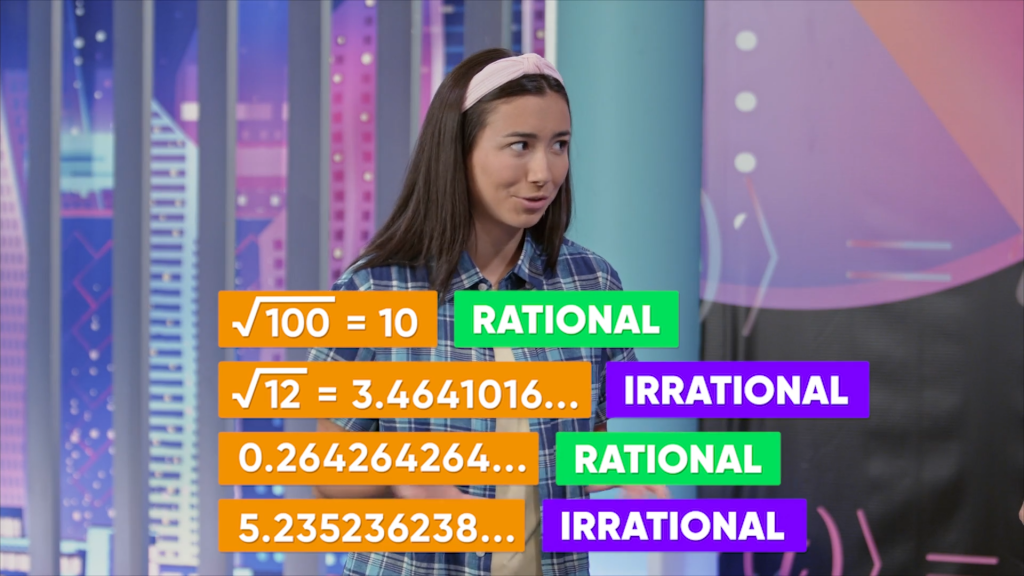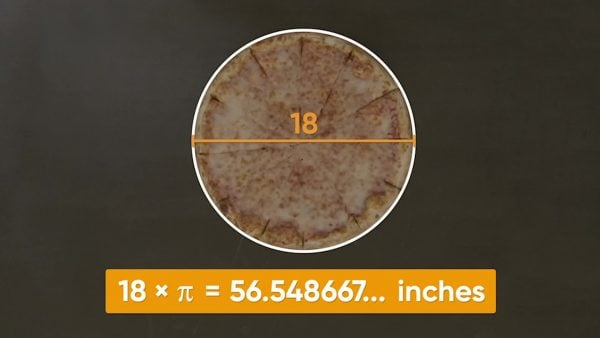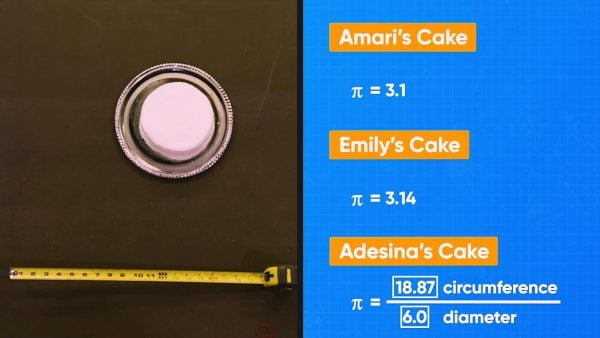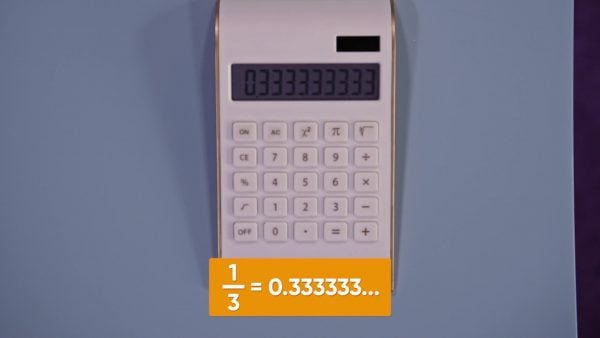Irrational Numbers Math Video for Kids - Grades 6-8
1%
It was processed successfully!WHAT IS AN IRRATIONAL NUMBER?

Rational numbers are numbers that can be represented with fractions (or ratios). Irrational numbers are numbers that cannot be represent with fractions. “Irrational” means “non-rational.”

To better understand irrational numbers…

WHAT IS AN IRRATIONAL NUMBER?. Rational numbers are numbers that can be represented with fractions (or ratios). Irrational numbers are numbers that cannot be represent with fractions. “Irrational” means “non-rational.” To better understand irrational numbers…

## LET’S BREAK IT DOWN!

### π is an irrational number.Adesina, Emily, and Amari are eating pizza. Amari wants to find the length of crust on their pizza. You can use the formula C = πd to find the circumference of the pizza, where C is the circumference and d is the diameter. The diameter is the distance across the center of the circle. The pizza has diameter 18 inches. The circumference of the pizza crust is C = πd = π(18) =56.54866776461628… The reason there are so many decimal places when you work with π is because π represents an irrational number. Try this yourself: Calculate the circumference of a circle that has diameter 17 inches.

π is an irrational number. Adesina, Emily, and Amari are eating pizza. Amari wants to find the length of crust on their pizza. You can use the formula C = πd to find the circumference of the pizza, where C is the circumference and d is the diameter. The diameter is the distance across the center of the circle. The pizza has diameter 18 inches. The circumference of the pizza crust is C = πd = π(18) =56.54866776461628… The reason there are so many decimal places when you work with π is because π represents an irrational number. Try this yourself: Calculate the circumference of a circle that has diameter 17 inches.

### You can use known measurements to calculate π.Adesina, Amari, and Emily are decorating cakes, and exploring the value of π. π is the ratio between the diameter of a circle and its circumference, π = [ggfrac]C/d[/ggfrac]. To determine the value of π, they decide to measure the circumference and diameter of their cakes and use their ratio to calculate π. Amari measures her cake and finds that the cake is 31 inches around and 10 inches across. She finds that the value of π is π = [ggfrac]C/d[/ggfrac] = [ggfrac]31/10[/ggfrac] = 3.1. Emily uses a more accurate tape measure on her cake and finds that the cake is 15.7 inches around and 5 inches in diameter. She finds that the value of π is π = [ggfrac]C/d[/ggfrac] = [ggfrac]15.7/5[/ggfrac] = 3.14. Next, Adesina measures her cake using an even more accurate tape measure. Adesina’s cake is 18.87 inches around and 6 inches in diameter, so her value of π is π = [ggfrac]C/d[/ggfrac] = [ggfrac]18.87/6[/ggfrac] = 3.145. All of their answers are close, but the varying accuracy of their measurements give them values for π with different levels of accuracy. If they used more precise scientific equipment, then they would have found π = π=3.14159265358979… The decimals in the number π go on forever and never repeat, which means it is an irrational number. Irrational numbers have decimals that go on forever and never repeat. We use the symbol π because it is a much more compact way to represent this irrational number. Try this yourself: Calculate π for a circle with circumference 62.8319 inches and diameter 20 inches.

You can use known measurements to calculate π. Adesina, Amari, and Emily are decorating cakes, and exploring the value of π. π is the ratio between the diameter of a circle and its circumference, π = [ggfrac]C/d[/ggfrac]. To determine the value of π, they decide to measure the circumference and diameter of their cakes and use their ratio to calculate π. Amari measures her cake and finds that the cake is 31 inches around and 10 inches across. She finds that the value of π is π = [ggfrac]C/d[/ggfrac] = [ggfrac]31/10[/ggfrac] = 3.1. Emily uses a more accurate tape measure on her cake and finds that the cake is 15.7 inches around and 5 inches in diameter. She finds that the value of π is π = [ggfrac]C/d[/ggfrac] = [ggfrac]15.7/5[/ggfrac] = 3.14. Next, Adesina measures her cake using an even more accurate tape measure. Adesina’s cake is 18.87 inches around and 6 inches in diameter, so her value of π is π = [ggfrac]C/d[/ggfrac] = [ggfrac]18.87/6[/ggfrac] = 3.145. All of their answers are close, but the varying accuracy of their measurements give them values for π with different levels of accuracy. If they used more precise scientific equipment, then they would have found π = π=3.14159265358979… The decimals in the number π go on forever and never repeat, which means it is an irrational number. Irrational numbers have decimals that go on forever and never repeat. We use the symbol π because it is a much more compact way to represent this irrational number. Try this yourself: Calculate π for a circle with circumference 62.8319 inches and diameter 20 inches.

### Square roots of numbers can be irrational.Adesina and the kids are trying to find the length of the long side in a right triangle. The triangle has short side lengths 3 feet and 4 feet. They can use the Pythagorean theorem to find the value of the missing side. The formula is c2 = a2 + b2 , where a and b are the lengths of the short sides. To find the value of c in the triangle, plug in the lengths of the sides for a and b: c2 = a2 + b2, c2 = 32 + 42, c2 = 5. So, the length of the longest side is 5 feet. Another triangle has two short sides with length 1 foot. The Pythagorean theorem says that the missing side length is √2. You can calculate this value using the square root button on your calculator. Adesina's calculator gives 1.414213562… This number is irrational because the decimal never terminates or repeats. In fact, the square roots of non-perfect squares are always irrational. Try this yourself: Identify whether each square root is rational or irrational. √6, √36, √17.

Square roots of numbers can be irrational. Adesina and the kids are trying to find the length of the long side in a right triangle. The triangle has short side lengths 3 feet and 4 feet. They can use the Pythagorean theorem to find the value of the missing side. The formula is c2 = a2 + b2 , where a and b are the lengths of the short sides. To find the value of c in the triangle, plug in the lengths of the sides for a and b: c2 = a2 + b2, c2 = 32 + 42, c2 = 5. So, the length of the longest side is 5 feet. Another triangle has two short sides with length 1 foot. The Pythagorean theorem says that the missing side length is √2. You can calculate this value using the square root button on your calculator. Adesina's calculator gives 1.414213562… This number is irrational because the decimal never terminates or repeats. In fact, the square roots of non-perfect squares are always irrational. Try this yourself: Identify whether each square root is rational or irrational. √6, √36, √17.

### Repeating and terminating decimals are rational.Emily converts [ggfrac]1/3[/ggfrac] to a decimal and gets 0.333333333… Is [ggfrac]1/3[/ggfrac] irrational? The decimal is endless, but the 3 in the decimal part repeats, so 13 is not an irrational number; it is a rational number. Rational numbers are numbers that can be written as a ratio of integer values. Their decimal form either terminates or repeats. You can summarize a repeating decimal by putting a bar across the repeated values, so 0.33333333333 becomes 0. 3. Emily then finds the decimal form of [ggfrac]2/3[/ggfrac] and gets 0.66666666, or 0.6. Likewise, [ggfrac]34/99[/ggfrac] becomes 0.3434343434, or 34. These are all rational numbers. Try this yourself: Identify whether each number is rational or irrational. 0.94

Repeating and terminating decimals are rational. Emily converts [ggfrac]1/3[/ggfrac] to a decimal and gets 0.333333333… Is [ggfrac]1/3[/ggfrac] irrational? The decimal is endless, but the 3 in the decimal part repeats, so 13 is not an irrational number; it is a rational number. Rational numbers are numbers that can be written as a ratio of integer values. Their decimal form either terminates or repeats. You can summarize a repeating decimal by putting a bar across the repeated values, so 0.33333333333 becomes 0. 3. Emily then finds the decimal form of [ggfrac]2/3[/ggfrac] and gets 0.66666666, or 0.6. Likewise, [ggfrac]34/99[/ggfrac] becomes 0.3434343434, or 34. These are all rational numbers. Try this yourself: Identify whether each number is rational or irrational. 0.94

## IRRATIONAL NUMBERS VOCABULARY

Whole number
Any of the numbers {0, 1, 2, 3, …}. They have no decimal part and do not include negative numbers.
Integer
Any of the numbers {…–3, –2, –1, 0, 1, 2, 3, …}. They have no decimal part and include both positive and negative numbers.
Terminating decimal
A decimal with a finite number of decimal places.
Repeating decimal
A decimal that continues forever but whose digits repeat.
Non-terminating decimals
A decimal that continues forever and whose digits do not repeat.
Irrational number
A number whose decimal form is non-terminating.
A number that can be represented by a fraction or integer ratio. The decimal form of a rational number is always a terminating or repeating decimal.
A special constant used to calculate the area and circumference of circles.
The square root of a number, n, is a number that, when multiplied by itself, has a product of n.

## IRRATIONAL NUMBERS DISCUSSION QUESTIONS

### What is a rational number?

A rational number is a number that can be written as a fraction of two integers. In decimal form, the decimals either terminate or form a repeating pattern.

### What is an irrational number?

An Irrational number is one whose decimal form never terminates and never repeats. They include special numbers like π, φ, or e, as well as square roots of non-perfect squares.

### If an irrational number has infinitely many decimal places, how can I possibly use it?

In practice, I can approximate an irrational number with a rational number that has enough decimal places to be useful in the problem. For example, if I need to know roughly how big √2 is, I may round to 1 decimal place to get my rational approximation. If I am an engineer and I need to be very precise, I might round to 6 decimal places. It is not practical or possible to work with infinitely many decimal places.

### Sort the following irrational numbers from least to greatest by converting each one to a rational approximation. 3.758375839203…, √22, [ggfrac]√20/4[/ggfrac], 3√2

3.758375839203…≈3.76, √22≈4.69, [ggfrac]√20/4[/ggfrac]≈1.12, 3√2≈4.24, so the order from least to greatest is [ggfrac]√20/4[/ggfrac], 3.758375839203…, 3√2, √22.

### Show that any terminating decimal can be written as a fraction with a base-ten denominator.

Write the decimal as a fraction with denominator 1. Then multiply the numerator and denominator by a power of 10 until the numerator is an integer.

Example: [ggfrac]2.55676/1.00000[/ggfrac] = [ggfrac]2.55676x100,000/1.00000x100,000[/ggfrac] = [ggfrac]255,676/100,000[/ggfrac]
X

## Success

We’ve sent you an email with instructions how to reset your password.
Ok